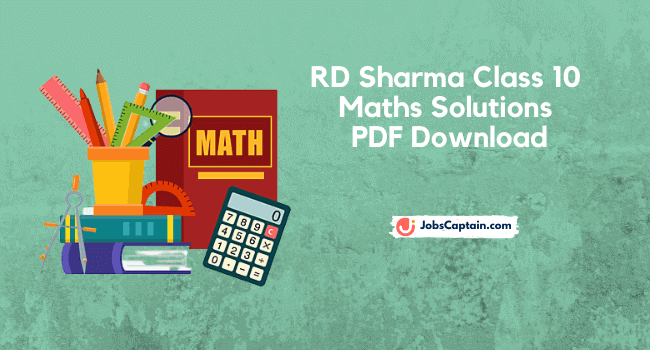By | April 9, 2021RD Sharma Maths Solutions Class 10 PDF is based on the lessons given in the CBSE textbook, so that students can learn specific concepts related to Maths subject. Using RD Sharma solutions, students can get knowledge of all principles an solve exercise wise Mathematics problems.

RD Sharma Maths Solutions Class 10 PDF is designed according to the NCERT guidelines. Class 10 Maths NCERT Book Pdf is explain Algebra, Geometry and Trigonometry, which are very important topics of mathematics.

Class 10 RD Sharma Maths Trigonometry is helps the students to gain conceptual knowledge because it provides an algorithmic approach. This class 10 maths solutions include all maths questions with detailed explanations.

The main purpose of RD sharma Maths book is to help students to self analyze their weaknesses and solve higher difficulty level problems in efficient manner.

You can download class 10 Maths solution RD sharma from here by clicking on below given buttons.

### RD Sharma Chapter-wise Maths Solutions

Chapter 1: Real Number
Exercise 1.1Exercise 1.2Exercise 1.3Exercise 1.4Exercise 1.5

Chapter 2: Polynomials
Exercise 2.1Exercise 2.2Exercise 2.3

Chapter 3: Pair of Linear Equations in Two Variables
1. Real Number
2. Polynomials
3. Pair of Linear Equations in Two Variables
4. Triangles
5. Trigonometric Ration
6. Trigonometric Identities
7. Statistics
9. Arithmetic Progressions
10. Circles
11. Constructions
12. Some Applications of Trigonometry
13. Probability
14. Coordinate Geometry
15. Areas Related to Circles
16. Surface Areas and Volumes

### Key Features of RD Sharma Maths Book

• Students can practice different types of questions and verify their difficulty level.
• By Referring to this Book Pdf, students can know the different means of solving questions and choose a suitable way.
• Maths solution also helps the students to encourage them and make learning process fast by practicing questions.

Thank you reading online or downloading Maths solutions by R D Sharma sir. Further more if you need any Maths solutions, then please do comment below. Our team will try best for you.

Category: Class 10 Tags: